## Perform ARIMA Model Residual Diagnostics Using Econometric Modeler App

This example shows how to evaluate ARIMA model assumptions by performing residual diagnostics in the Econometric Modeler app. The data set, which is stored in `Data_JAustralian.mat`, contains the log quarterly Australian Consumer Price Index (CPI) measured from 1972 and 1991, among other time series.

### Import Data into Econometric Modeler

At the command line, load the `Data_JAustralian.mat` data set.

`load Data_JAustralian`

At the command line, open the Econometric Modeler app.

`econometricModeler`

Alternatively, open the app from the apps gallery (see Econometric Modeler).

Import `DataTimeTable` into the app:

1. On the Econometric Modeler tab, in the Import section, click the button.

2. In the Import Data dialog box, in the Import? column, select the check box for the `DataTimeTable` variable.

3. Click .

The variables, including `PAU`, appear in the Time Series pane, and a time series plot containing all the series appears in the Time Series Plot(EXCH) figure window.

Create a time series plot of `PAU` by double-clicking `PAU` in the Time Series pane.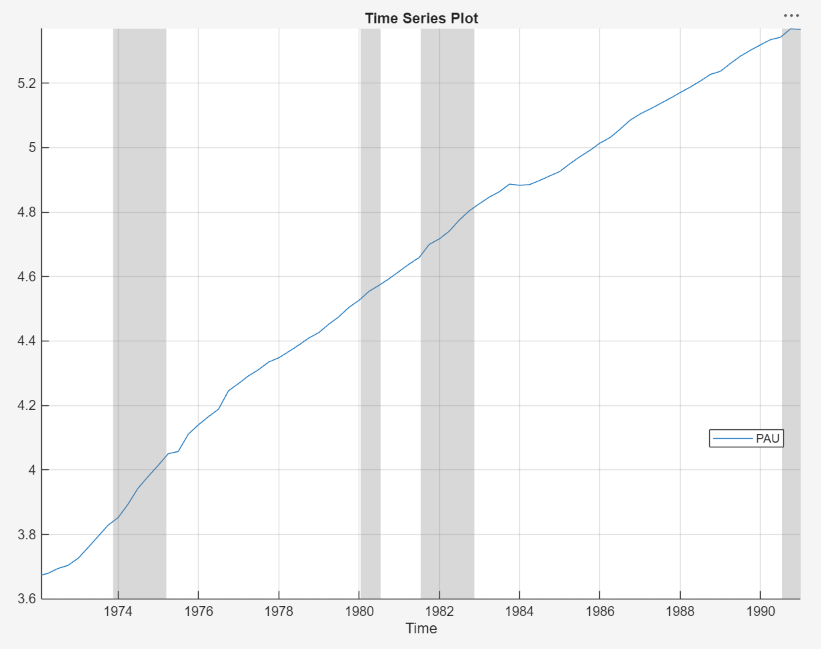### Specify and Estimate ARIMA Model

Estimate an ARIMA(2,1,0) model for the log quarterly Australian CPI (for details, see Implement Box-Jenkins Model Selection and Estimation Using Econometric Modeler App).

1. In the Time Series pane, select the `PAU` time series.

2. On the Econometric Modeler tab, in the Models section, click ARIMA.

3. In the ARIMA Model Parameters dialog box, on the Lag Order tab:

1. Set the Degree of Integration to `1`.

2. Set the Autoregressive Order to `2`.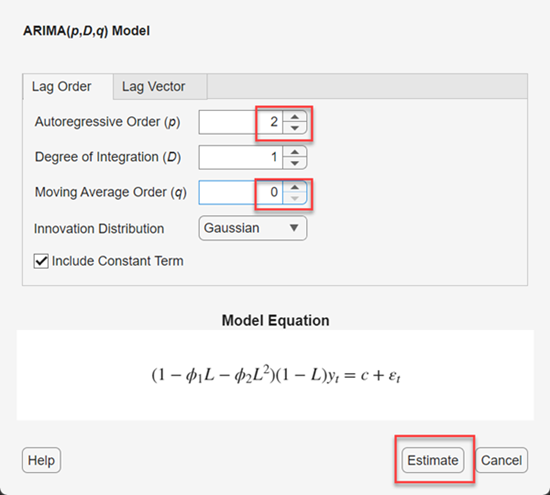4. Click .

The model variable `ARIMA_PAU` appears in the Models pane, its value appears in the Preview pane, and its estimation summary appears in the Model Summary(ARIMA_PAU) document.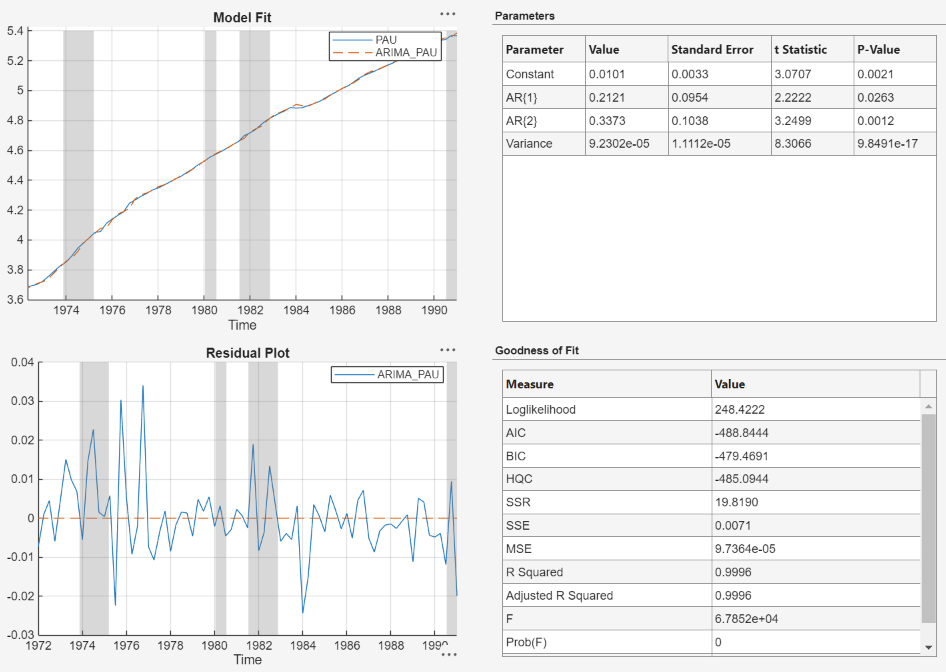In the Model Summary(ARIMA_PAU) document, the Residual Plot figure is a time series plot of the residuals. The plot suggests that the residuals are centered at y = 0 and they exhibit volatility clustering.

### Perform Residual Diagnostics

Visually assess whether the residuals are normally distributed by plotting their histogram and a quantile-quantile plot:

1. Close the Model Summary(ARIMA_PAU) document.

2. With `ARIMA_PAU` selected in the Models pane, on the Econometric Modeler tab, in the Diagnostics section, click > Residual Histogram.

3. Click > Residual Q-Q Plot.

Inspect the histogram by clicking the Histogram(ARIMA_PAU) figure window.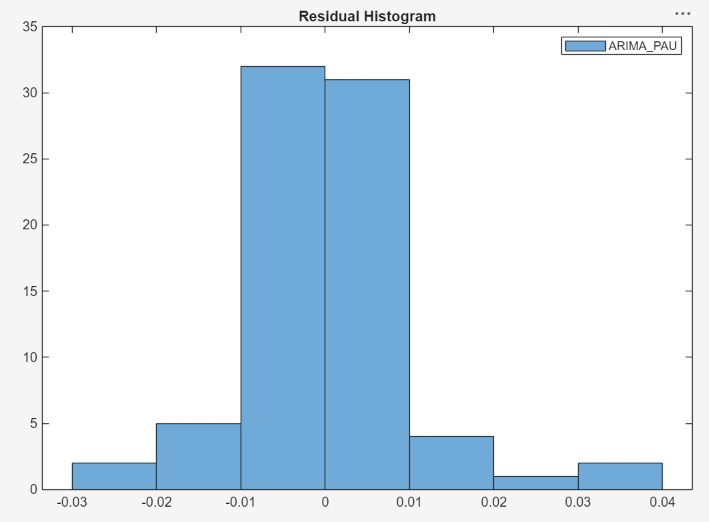Inspect the quantile-quantile plot by clicking the QQPlot(ARIMA_PAU) figure window.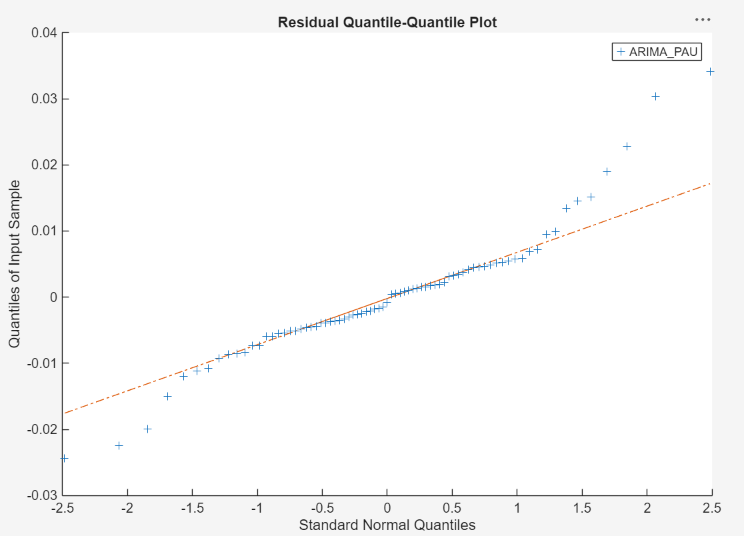The residuals appear approximately normally distributed. However, there is an excess of large residuals, which indicates that a t innovation distribution might be a reasonable model modification.

Visually assess whether the residuals are serially correlated by plotting their autocorrelations. With `ARIMA_PAU` selected in the Models pane, in the Diagnostics section, click > Autocorrelation Function.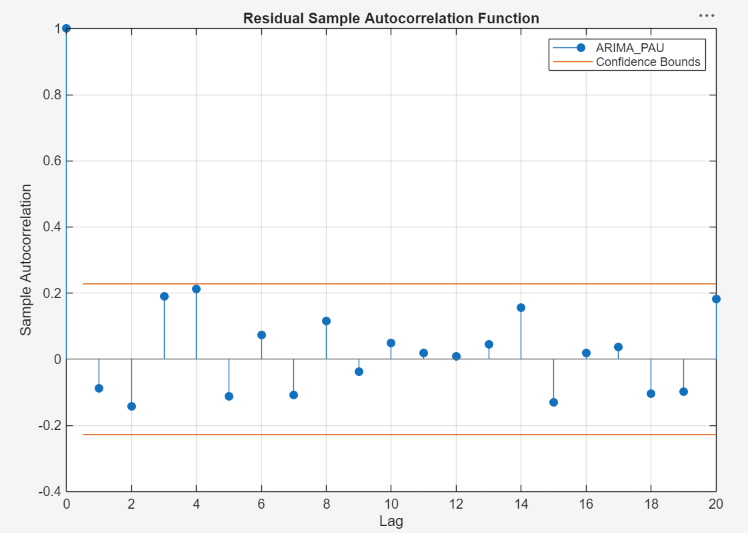All lags that are greater than 0 correspond to insignificant autocorrelations. Therefore, the residuals are uncorrelated in time.

Visually assess whether the residuals exhibit heteroscedasticity by plotting the ACF of the squared residuals. With `ARIMA_PAU` selected in the Models pane, click the Econometric Modeler tab. Then, click the Diagnostics section, click > Squared Residual Autocorrelation.Significant autocorrelations occur at lags 4 and 5, which suggests a composite conditional mean and variance model for `PAU`.